International Journal of Astrophysics and Space Science
Volume 3, Issue 4, August 2015, Pages: 55-59

Nonplanar Geometry Dust – Ion Acoustic Shocks in an Adiabatic Dusty Plasma with Nonthermal Electrons

Louis E. Akpabio*, Akaninyene D. Antia

Theoretical physics Group, Department of Physics, University of Uyo, Uyo, Nigeria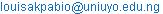(L. Akpabio)

Louis E. Akpabio, Akaninyene D. Antia. Nonplanar Geometry Dust – Ion Acoustic Shocks in an Adiabatic Dusty Plasma with Nonthermal Electrons. International Journal of Astrophysics and Space Science. Vol. 3, No. 4, 2015, pp. 55-59. doi: 10.11648/j.ijass.20150304.11

Abstract: An unmagnetized dusty plasma consisting of static negatively charged dust fluid, nonthermal distributed electrons, and adiabatic ion fluid has been considered. Basic properties of the dust-ion-acoustic shock waves have been made by the reductive perturbation method to derive the Burgers’ equation for nonplanar geometry. The solution of modified Burgers’ equation in nonplanar geometry is numerically analyzed and it has been found that, the nonplanar geometry effects have a very vital role in the development of shock waves. We also discovered that; the inclusion of the nonthermal electron distribution significantly modifies the shock wave profile. The change of the DIASW structure due to the effect of ion temperature and dust density is studied.

Keywords: Nonplaner Geometry, Dust –Ion Acoustic Shocks, Adiabatic Dusty Plasma, Non-Thermal Electron

1. Introduction

The interplay between charged dust grain and plasmas has lead to much interest in new research area called dusty (or complex) plasma. Linear as well as nonlinear collective processes in dusty or complex plasma have received special attention due to the realization of their occurrence in planetary rings, interstellar clouds and cometary environment [1-7]. There has been a rapidly growing interest in nonlinear phenomena (such as shocks, solitons and vortices) in dusty plasma (plasmas with extremely massive and negatively charged dust grains), because of its crucial role in understanding electrostatic disturbances in space  and laboratory dusty plasmas [9-13]. Now, it is a well known fact that the presence of static charged dust grains modifies the existing plasma wave spectra [14-16].

Dust ion acoustic shocks in unmagnetized dusty plasma may arise when there is a balance between the nonlinearity (associated with the harmonic generation) and the kinematics viscosity, introduced by the dust ion drag. The formation of dust ion acoustic shock wave (DIASWs) was observed by Nakamura et al. . They found out that in the linear regime, the phase velocity of the Dust Ion Acoustic (DIA) waves increases while the wave suffers heavy damping when the dust number density in an electron –ion plasma is considerably increased. On the other hand, they found out that an oscillatory ion-acoustic shock wave in usual argon plasma transforms into a monotonic shock front when it travels through the dusty plasma column. A number of studies have been made on the propagation of DIASWs in dusty plasma by several investigators [18 – 21].

Recently, several theoretical investigations [22 – 26] on the properties of dust-ion acoustic and dust acoustic solitary waves in nonplanar geometry had been carried out. Likewise, of recent, a theoretical investigation has been done by Sahu  to study the effect of nonplanar DIASWs in adiabatic dusty Plasma. Moreover, in real dusty plasma, the effect of finite ion temperature cannot be neglected and the electron behaviour can be strongly modified by nonlinear potential of the localized DIA structures by generating a population of fast energetic electrons. Therefore; in our present paper, we wish to study how finite ion temperature combined with electron non-thermality effects can be expected to modify the results arrived at in Sahu . The manuscript is organized as follows; section 2 deals with the basic equations governing the adiabatic dusty plasma system under consideration, section 3 presents the investigation of nonplanar DIASWs by the reduction perturbation method (RPM). In section 4, we present the numerical results and discussion, while section 5 is the conclusion.

2. Governing Equations

We consider an unmagnetized plasma comprising static negatively charged dust fluid, nonthermal distributed electrons and adiabatic ion fluid. The dynamics of the DIASWs in nonplanar geometry for such dusty plasma is governed by the following normalized fluid equations: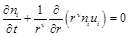(1)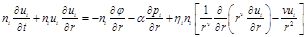(2)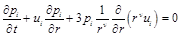(3)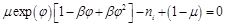(4)

whereis a parameter determining the number of non thermal electrons present in our plasma model. In the above equation,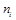is the ion number density normalized by its equilibrium value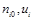is the ion fluid speed normalized by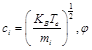is the wave potential normalized by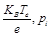is the ion thermal pressure normalized by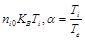. The time and space variables are normalized by reciprocal plasma frequency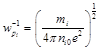and the Debye length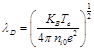respectively. While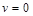, for one-dimensional geometry,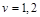for cylindrical and spherical geometry respectively.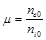and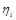is the viscosity coefficient of ion fluid normalized by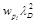. Note that in equation (4), we assumed the quasi-neutrality condition.

3. Derivation of Nonplanar Burgers’ Equation

We derive the Burgers’ equation from equations (1) – (4) by employing the reductive perturbation method (RPM)  and the stretched coordinates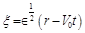and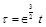, where Î is a smallness parameter measuring the weakness of the nonlinearity and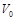is the phase speed of DIASWs normalized by. Equations (1) – (4) can be expressed in terms of x and t as follows: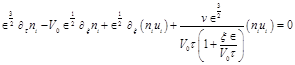(5)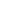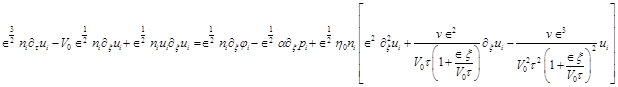(6)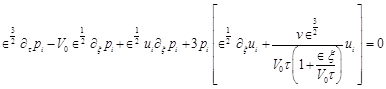(7)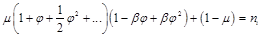(8)

where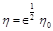is assumed . We can expand the dependent variables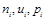and f in power series of Î as follows: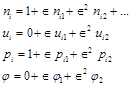(9)

Now, substituting equation (9) into equations (5) – (8), we obtain the lowest order of the coefficient of Î as: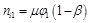(10)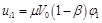(11)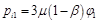(12)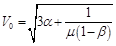(13)

The next higher order in Î, is given as the following set of equations,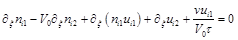(14)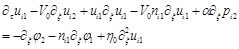(15)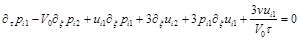(16)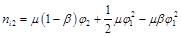(17)

Now, using equations (10) – (17) and eliminatingand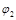, we finally obtain a modified Burgers’ equation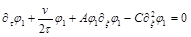(18)

where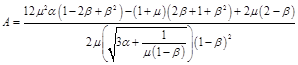(19)(20)

4. Numerical Results and Discussion

Equation (18) is the modified Burgers’ equation describing the nonlinear propagation of the DIASWs in unmagnetized dusty plasma consisting of adiabatic ion fluid, nonthermal distributed electrons and static negatively charged dust fluid. The stationary DIASWs of this modified Burgers’ equation for planar geometry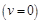is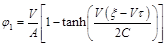Where V is a constant velocity normalized by c­­i. For nonplanar geometry, an exact analytical solution of equation (18) is not possible, hence it is solved numerically.

Figure 1, shows the variation of shock wave structure with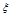for the different given parameters in different geometries. It is interesting to point out that the inclusion of non-thermal electron distribution gets the shock profile modified in terms of the shock height and shock steepness for the different geometry. The height and steepness of one-dimensional shock wave are larger than that of cylindrical shock wave, which in turn is larger than that of the spherical shock wave. The shock height is found to be decreasing monotonically with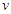(the geometry).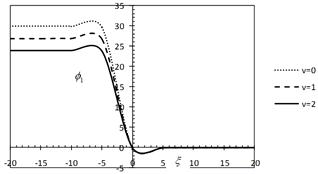Fig. 1. Showing how the shock profile (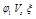curves) varies in different geometries for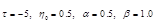and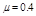.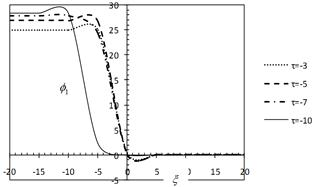Fig. 2. Variation of f1 with respect to x at different values of t for the cylindrical geometry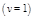and the other parameters being the same as figure 1.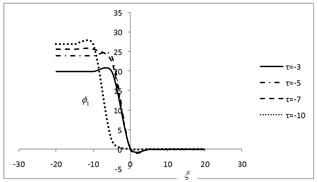Fig. 3. Variation of f1 with respect to x at different values of t for the spherical geometry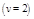and the other parameters being the same as figure 1.

In figures 2 and 3, we present the effect of several values of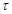for both cylindrical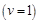and spherical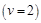geometries respectively. It can be observed that as the value ofincreases, the shock wave structures look like that of the one-dimensional geometry of fig. 1. This is due to the fact that the nonplanar geometrical effect is no longer prominent for larger values of. The nonplanar geometrical effects given by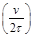will become effective as the value of t decreases and the shock wave profiles differs from each other in both cylindrical and spherical geometry respectively.

The shock wave structures for different values of the nonthermal electron parameter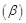are investigated in both cylindrical and spherical geometries as shown in figures 4 and 5 respectively. It is found that; small variation, in the magnitude of b significantly affects the shock wave profile. The shock height is found to be increasing monotonically with b. This effect is more pronounced in cylindrical geometry when compared to the spherical geometry.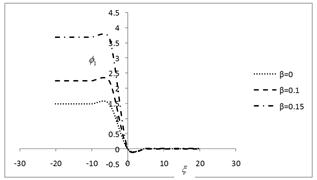Fig. 4. Variation of f1 with respect to x at different values of nonthermal electron parameter (b) for the cylindrical geometryand the other parameters being the same as figure 1.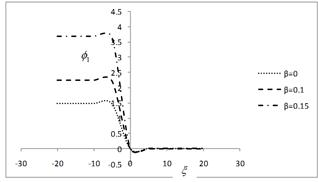Fig. 5. Variation of f1 with respect to x at different values of nonthermal electron parameter (b) for the spherical geometryand the other parameters being the same as figure 1.

The effects of ion temperature (a) and dust density (m) on the shock wave structures are also studied. The dust density can be expressed by the variation of m owing to 1- m = Zdndo/ nio . Figure 6 shows how the steepness of the shock wave structure is also modified by dust density and it decreases with dust density. While from fig. 7, it can be observed that the ion temperature has a weak effect on the steepness of shock wave structure.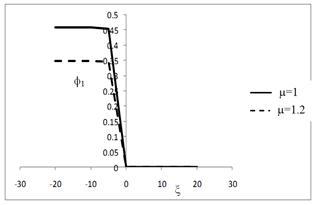Fig. 6. Variation of f1 with respect to x at different values of m for the cylindrical geometry (n=1) and the other parameters being the same as figure 1.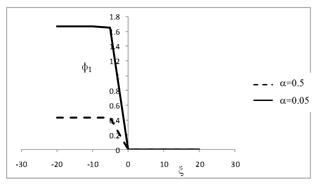Fig. 7. Variation of f1 with respect to x at different values of a(ion temperature) for the cylindrical geometry (n=1) and the other parameters being the same as figure 1.

5. Conclusion

We have derived the cylindrical and spherical Burgers’ equation for Dust-ion acoustic shock waves in unmagnetized adiabatic dusty plasmas comprising static negatively charged dust fluid, nonthermal distributed electrons and adiabatic ion fluid. Employing the standard reductive perturbation method to derive the Burgers’ nonplanar geometry equation, the choice of non-thermal distribution for energetic electrons used in our model is due to its convenience rather than as a precise fitting model of what is observed.

We have found out that, the propagation of Dust ion acoustic shock wave in nonplanar geometry with the inclusion of non-thermal electrons distribution gets the shock wave modified as the shock profile is found to vary significantly with the nonthermalparameter b. This effect is more prominent in cylindrical as compared to the spherical geometry. It is also observed that, the shock height decreases monotonically with the geometry. We realize that for large negative values of t, the nonplanar geometry approaches the planar geometry. Finally, the amplitude and steepness of the one-dimension shock waves are larger than that of the cylindrical shock waves, which in turn is larger than that of the spherical shock waves. we have also shown that the ion temperature and the dust density will modify the properties of DIASWs. These are also confirmed by experiments. This work could further throw more light on the understanding of the nonlinear propagation characteristic of DIASWs that are necessary in laboratory plasma as well as in plasma application.

References

1. Shukla, P.K.(2001). A Survey of dusty plasma physics. Phys. Plasmas 8(5): 1791-1803.
2. Bliokh, P., Sinitsin, V. and Yaroshenko, V. (1995) Dusty and Self-Gravitational Plasmas in Space. Kluwer Academic, Dordrecht.
3. Shukla, P.K., Mendis, D.A. and Chow, V.W. (1996). The Physics of Dusty Plasmas. World Scientific, Singapore.
4. Eya, I. O. and Urama, J. O. (2014). Statistical  Study of Neutron Star Glitches. Int. J. Astrophysics and Space Science 2(2): 16 -21.
5. Mendis, D.A. and Rosenberg, M. (1992). Some aspects of dusty-plasma interaction in cosmic environment IEEE Trans. Plasma Sci. 20(1): 929 – 934.
6. Mendis, D. A., Shukla, P.K., Mendis, D.A. and Desai, T. (1997). Advances in Dusty Plasmas. World Scientific, Singapore.
7. Verheest, F. (2000). Waves in Dusty Space Plasmas. Kluwer Academic, Dordrecht.
8. Abdul, K. and Duorah, K. (2015).  Electron-Positron Pairs Related to Alfven Waves on the Magnetar Surfaces. Int. J. Astrophysics and Space Science 3(3): 25 -29.
9. Geortz, C. K. (1989). Dusty Plasma in the solar system. Rev. Geophys. 27: 271.
10. Mendis, D.A. and Rosenberg, M. (1994). Cosmic Dusty Plasmas. Annu. Rev. Astrophys. 32: 419.
11. D’Angelo, N. (1990). Low frequency electrostatic waves in dusty Plasma Planet. Space Sci. 38 (9): 1142 – 1146.
12. Barkan, A., D’Angelo, N. and Merlino, R. L. (1994). Charging of Dust Grains in plasma. Phys. Rev. Lett. 73: 3093 – 3096.
13. Barkan, A., Merlino, R. L. and D’Angelo, N. (1995). Laboratory Observation of the dust-acoustic wave mode. Phys. Plasmas 2: 3563 – 3565.
14. Bliokh, P. V. and Yaroshenko, V. V. (1985). Electrostatic Waves in Saturn’s rings. Sov. Astron. 29: 330 – 336.
15. De Angelis, U., Formisano, V. and Giordano, M. (1988). Ion Plasma waves in dusty plasmas. Halley’s Comet. J. Plasma Phys. 40: 399 – 406.
16. Shukla, P. K. and Sihin, V. P. (1992). Dust ion-acoustic waves. Phys. Scripta 45: 508.
17. Nakamura, Y., Bailung, H. and Shukla, P. K. (1999). Observation of ion-acoustic shocks in a dusty plasmas. Phys. Rev. Lett. 83: 1602.
18. Shukla, P. K. (2000). Dust ion-acoustic shocks and holes. Phys. Plasmas 7: 1044.
19. Shukla, P. K. and Mamun, A. A. (2002) Introduction to Dusty Plasma Physics. Bristol: IOP Publishing Ltd.
20. Shukla, P. K. and Mamun, A.A. (2003). Solitons, Shocks and Vortices in dusty plasmas. New J. Phys. 5: 17.2 – 17.37.
21. Rahman, A., Sayed, F. and Mamun, A. A. (2007). Dust ion-acoustic shock waves in an adiabatic dusty Plasma. Phys Plasma 14: 034503.
22. Mamun, A. A. and Shukla, P. K. (2002). Solitary Potentials in cometry dusty Plasma. Geophys. Res. Lett. 29: 1870. doi:10.1029/2002 GLO15219.
23. Jukui, X. and He, L. (2003). Modulational instability of Cylindrical and spherical dust ion-acoust waves. Phys. Plasmas. 10: 339.
24. Xue, J. K. (2003). A Spherical KP equation for dust acoustic waves. Phy. Lett. A 314: 479.
25. Xue, J. K. (2003). Cylindrical dust acoustic waves with transverse perturbation. Phys. Plasmas 10: 3430.
26. Xue, J. K. (2005). Nonplanar dust ion-acoustic shock waves with transverse perturbation. Phys. Plasmas 2: 012314.
27. Sahu, B. (2011). Cylindrical or Spherical Dust ion Acoustic Shocks in an Adiabatic Dusty Plasma. Bulg. J. Phys. 38: 175 – 183.
28. Washimi, H. and Taniuti, T. (1996). Propagation of ion-acoustic solitary wave of small amplitude. Phys. Rev. Lett. 17: 996 – 998.

 Contents 1. 2. 3. 4. 5.
Article ToolsAbstractPDF(283K)